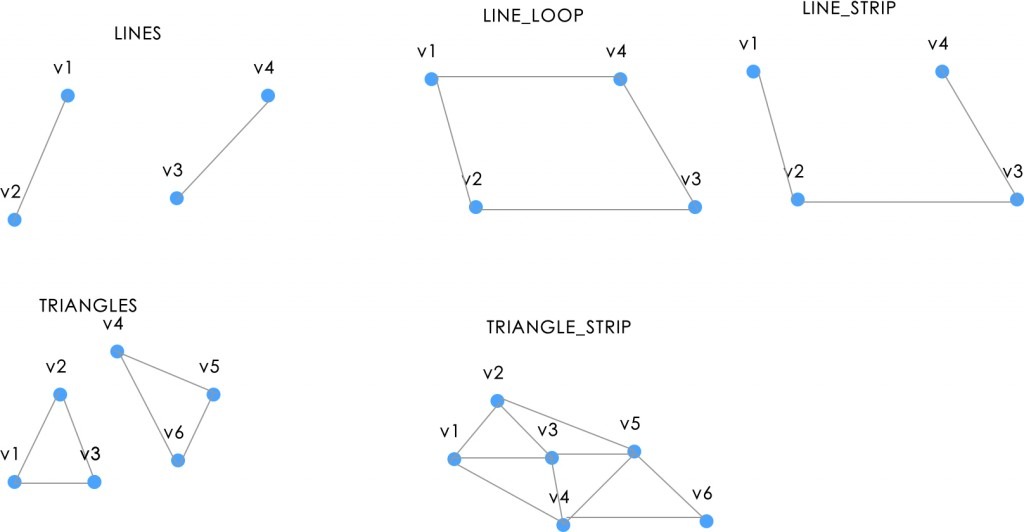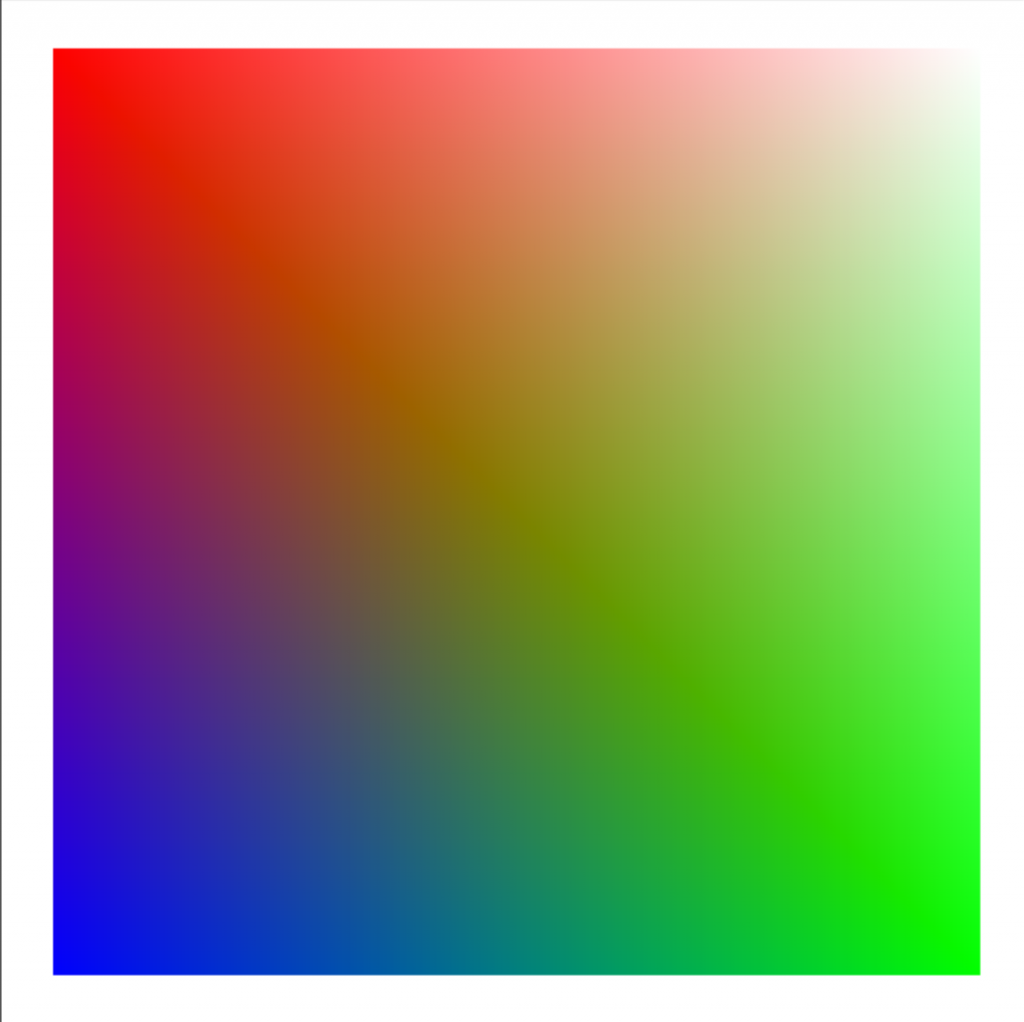#2017 iT 邦幫忙鐵人賽
DAY 6
0
Modern Web

# [Day6] webGL 修羅道(3) - Animation 與 varying

demo

• gl.POINTS：僅繪製頂點
• gl.LINES：從 buffer 中取兩點，並且繪製成線
• gl.LINE_STRIP：每個頂點按照順序連起來
• gl.LINE_LOOP：跟 LINE_STRIP 相同，但是最後一個頂點會連接回第一個頂點
• gl.TRIANGLES：每次取三個點繪製三角形
• gl.TRIAGLE_STRIP：從第三個頂點開始，每個頂點和前兩個連接
• gl.TRIANGLE_FAN：從第三個頂點開始，每個頂點和第一個和上一個頂點連接## Animation 簡介

demo2-布朗隨機運動

### 布朗隨機運動

``````function draw(gl, vertices) {

// 布朗隨機運動
for(var i = 0; i < POINTS_COUNT * 2; i += 2) {
vertices[i] += Math.random() * 0.01 - 0.005;
vertices[i + 1] += Math.random() * 0.01 - 0.005;
}

gl.bufferData(gl.ARRAY_BUFFER, new Float32Array(vertices), gl.STATIC_DRAW);
gl.bufferSubData(gl.ARRAY_BUFFER, 0, new Float32Array(vertices));
gl.clear(gl.COLOR_BUFFER_BIT);
gl.drawArrays(gl.POINTS, 0, POINTS_COUNT);

const bindDraw = (gl, vertices) => () => draw(gl, vertices);
requestAnimationFrame(bindDraw(gl, vertices));
}
``````

gl.STATIC_DRAW 代表我們常常會用到 buffer 的資料，但不會更動它的值。

### 圓形分佈

``````var vertices = times(POINTS_COUNT * 2)
.map((val, i) => Math.sin(Math.random()) *- 0.01);
``````

## varying 應用

``````<script id="shader-fs" type="x-shader/x-fragment">
precision mediump float;
varying lowp vec4 vColor;
void main(void) {
gl_FragColor = vColor;
}
</script>
attribute vec4 position;
attribute float size;

attribute vec4 aVertexColor;

varying lowp vec4 vColor;

void main(void) {
gl_Position = position;
vColor = aVertexColor;
}
</script>
``````demo

### 來不及說完的事

• 紋理貼圖：我們前幾個範例當中，顏色幾乎都是基於頂點定義的。但更多時候，我們應該是將紋理放入我們的 3D 模型中。
• 光源計算、隱影：在 GSGL 中可以做光源計算，不過礙於篇幅的關係，這邊並不細講，到 `three.js` 的時候，我們再來深入了解光源。
• 深度測試與混合

## 學習資源

webGL 幾乎可以算是全新的領域，對於前端來說可以擴展更多可能性。以下列出一些有用的教學資源，其實我們 webGL 要在一個禮拜內全部講完是不太可能的，不過這幾天的課程應該具備基本的 webGL 知識了。

• webgl fundamental 教學很詳盡的網站，內容非常豐富，全部看完可以對 webGL 有更深入的了解
• learning webgl 另外一個 webgl 的教學網站，可以學到蠻豐富的東西。
• webgl 自學網 翻譯自 learning webgl 的英文網站。

### 1 則留言

0